## Linear Categories: A Folklore Simplification

Updated: 2018-07-24

Recently, I read this paper. The authors use a simplified definition of a linear category, but without proof that their definition is really the same. So I emailed them about it, and started a nice conversion with Damiano Mazza, Marco Gaboardi, and Flavien Breuvart. Then at FLOC 2018 this year I got the opportunity to meet Damiano Mazza, Marco Gaboardi, and a few others for lunch. I learned from Damiano that that their simplification to the definition of linear categories is correct, but this simplification seems to be folklore. So in this post I am going to write out this simplification, and prove that it is equivalent to the original definition of a linear category.

## Linear Categories

Linear categories are one of the first sound and complete categorical models of intuitionistic linear logic proposed in Gavin Bierman’s thesis. He shows that the linear exponential, $!A$, can be modeled as a symmetric monoidal comonad. His original definition (Definition 35 on p. 140) is as follows.

A linear category, $\cat{C}$, consists of the following structure:

1. A symmetric monoidal category, $\cat{C}$, with finite products and coproducts,

2. A symmetric monoidal comonad $(!,\varepsilon, \delta, \m_{A,B}, \m_I)$ such that

1. For every free $!$-coalgebra $(!A,\delta_A)$ there are two distinguished monoidal natural transformations with components:

• (Weakening) $\w_A : !A \mto I$
• (Contraction) $\c_A : !A \to !A \otimes !A$

which form a commutative comonad and are coalgebra morphisms.

2. Whenever $f : (!A,\delta_A) \mto (!B,\delta_B)$ is a coalgebra morphism between free coalgebras, then it is also a comonoid morphism.

There is a lot packed into this definition, but we will expanded it in the next section.

## Linear Categories Simplified

Essentially, the simplification amounts to realizing that part b can be proven from the previous parts of the definition of a linear category, but with an additional assumption that is simpler than part b. The expanded simplified definition is as follows.

A linear category, $\cat{A}$, consists of the following structure:

1. A is a symmetric monoidal category $(\mathcal{A},\otimes,I,\lambda,\rho,\alpha,\beta)$,

2. A linear exponential comonad, $(!_{-},\delta,\varepsilon)$, which has the following structure:

• The endofunctor $! : \mathcal{A} \mto \mathcal{A}$ forms comonad on $\cat{A}$. That is, there are two natural transformations $\delta : ! A \mto !! A$ and $\varepsilon : !A \mto A$ that make the following diagrams commute: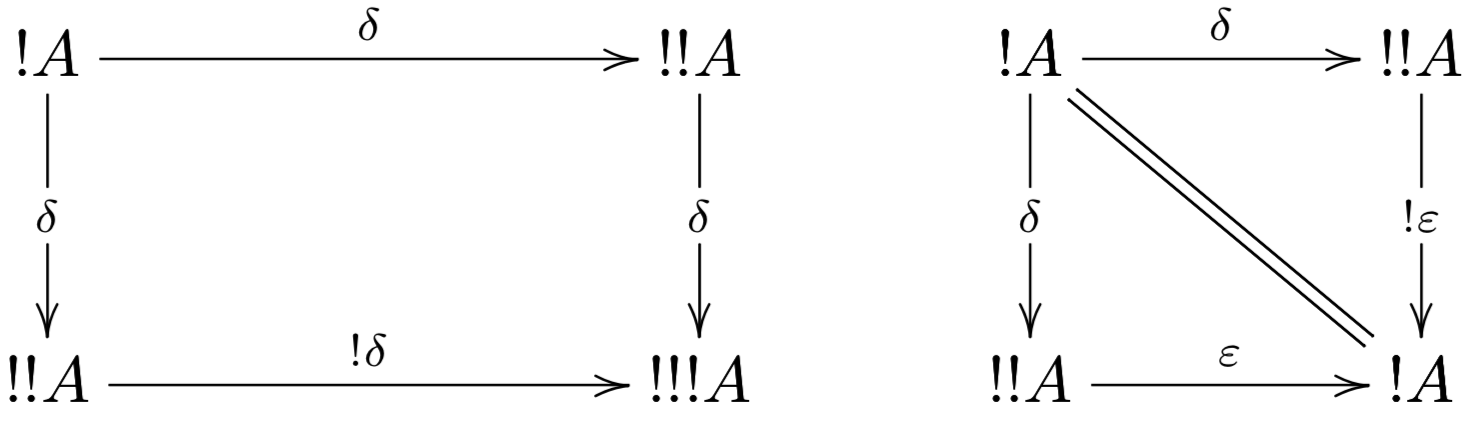• Four natural transformations:

• (Monoidal Map) $\m_{A,B} : ! A \otimes ! B \mto ! (A \otimes B)$

• (Monoidal Unit Map) $\m_I : I \mto ! I$

• (Contraction) $\c : !A \mto !A \otimes !A$

• (Weakening) $\w : ! A \mto I$

• The functor $! : \mathcal{A} \mto \mathcal{A}$ is symmetric monoidal. That is, the following diagrams must commute: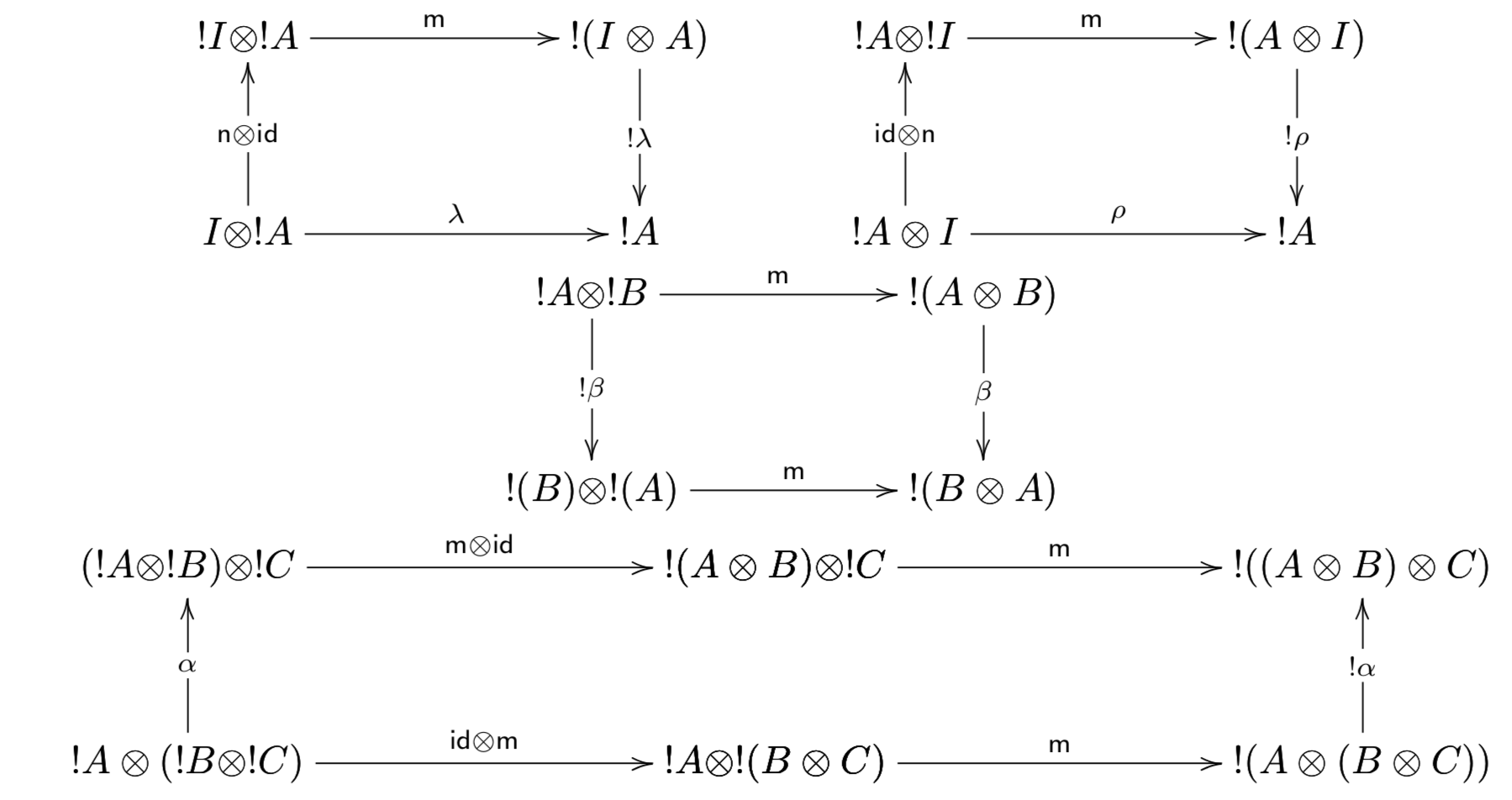• The functor $! : \cat{A} \mto \cat{A}$ is a symmetric monoidal comonad. That is, the following diagrams must commute: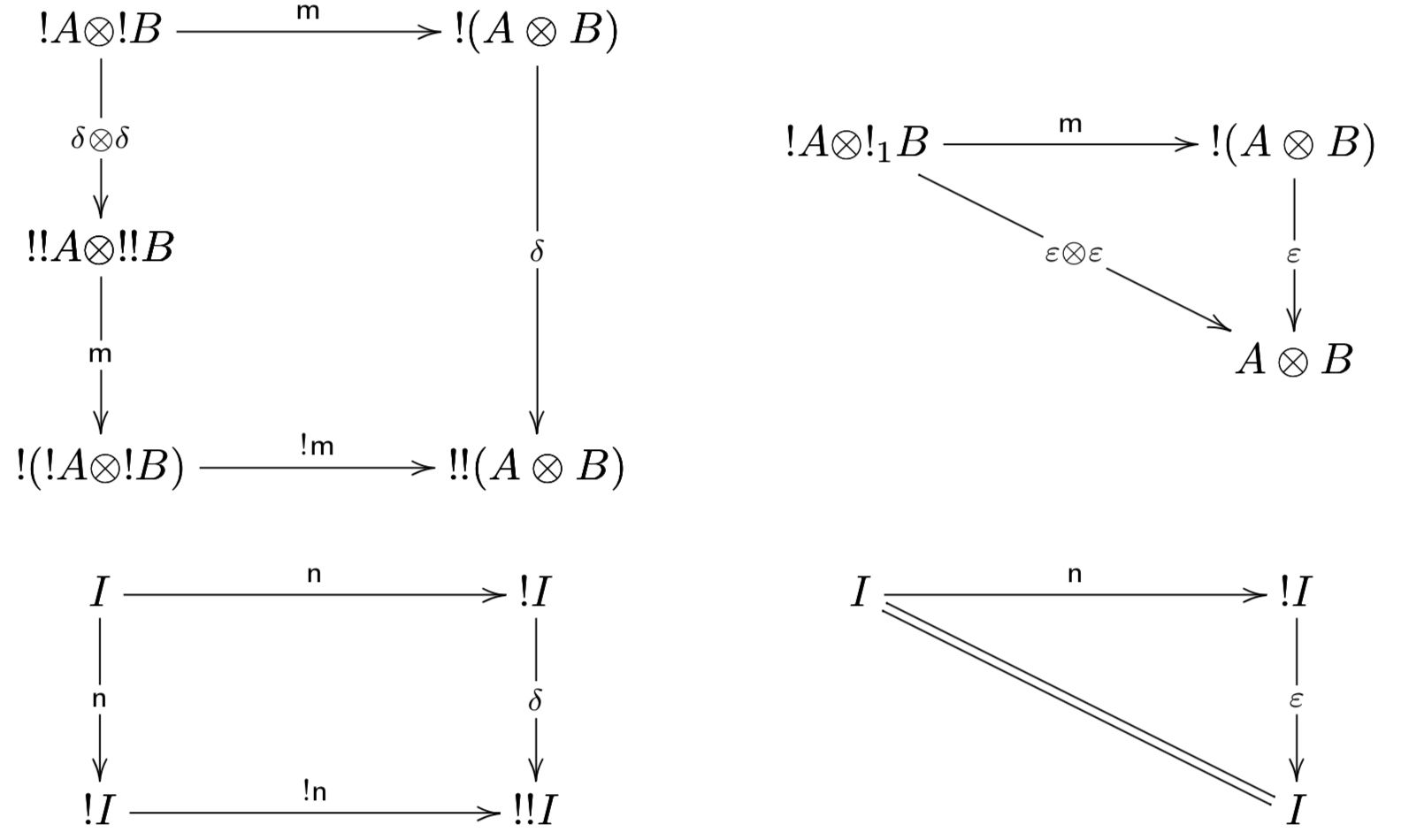• Weakening must satisfy the following diagrams: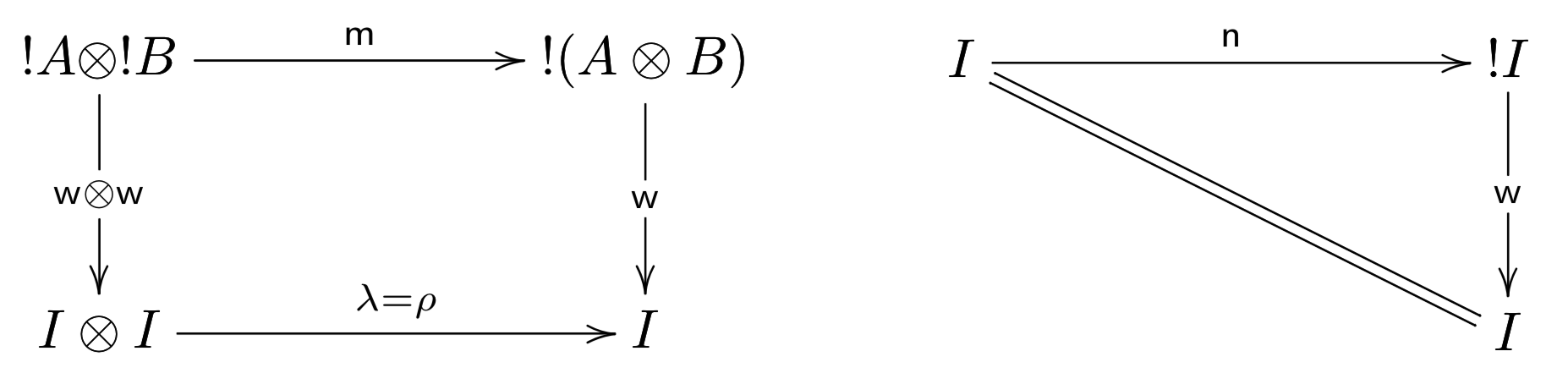• Contraction must satisfy the following diagrams: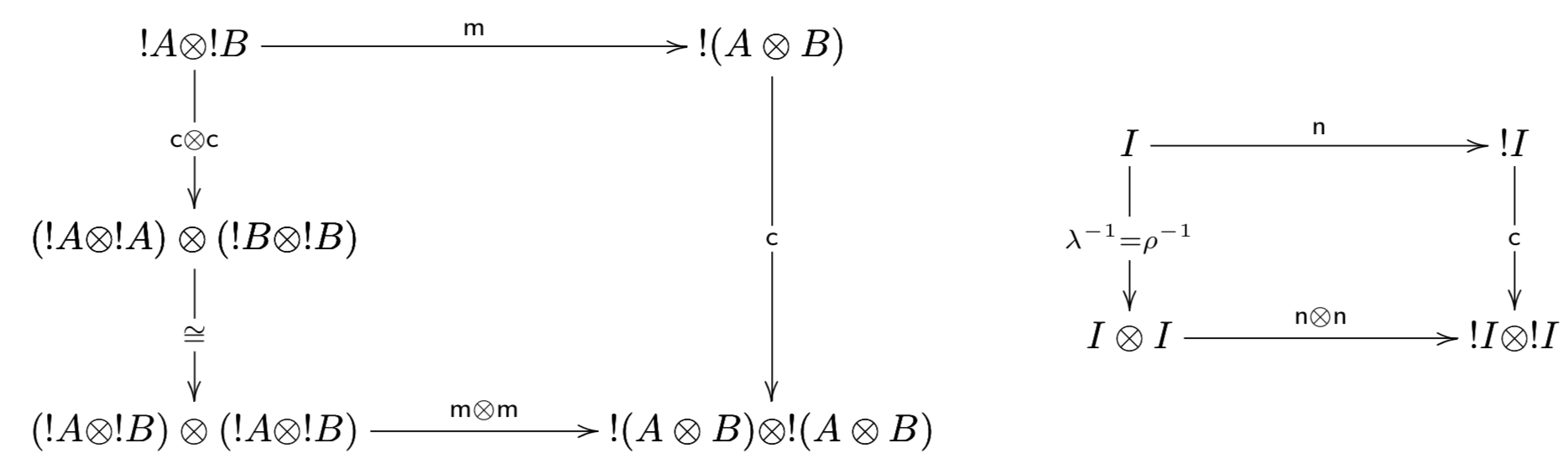• Weakening and contraction form a commutative comonoid. That is, the following diagrams commute: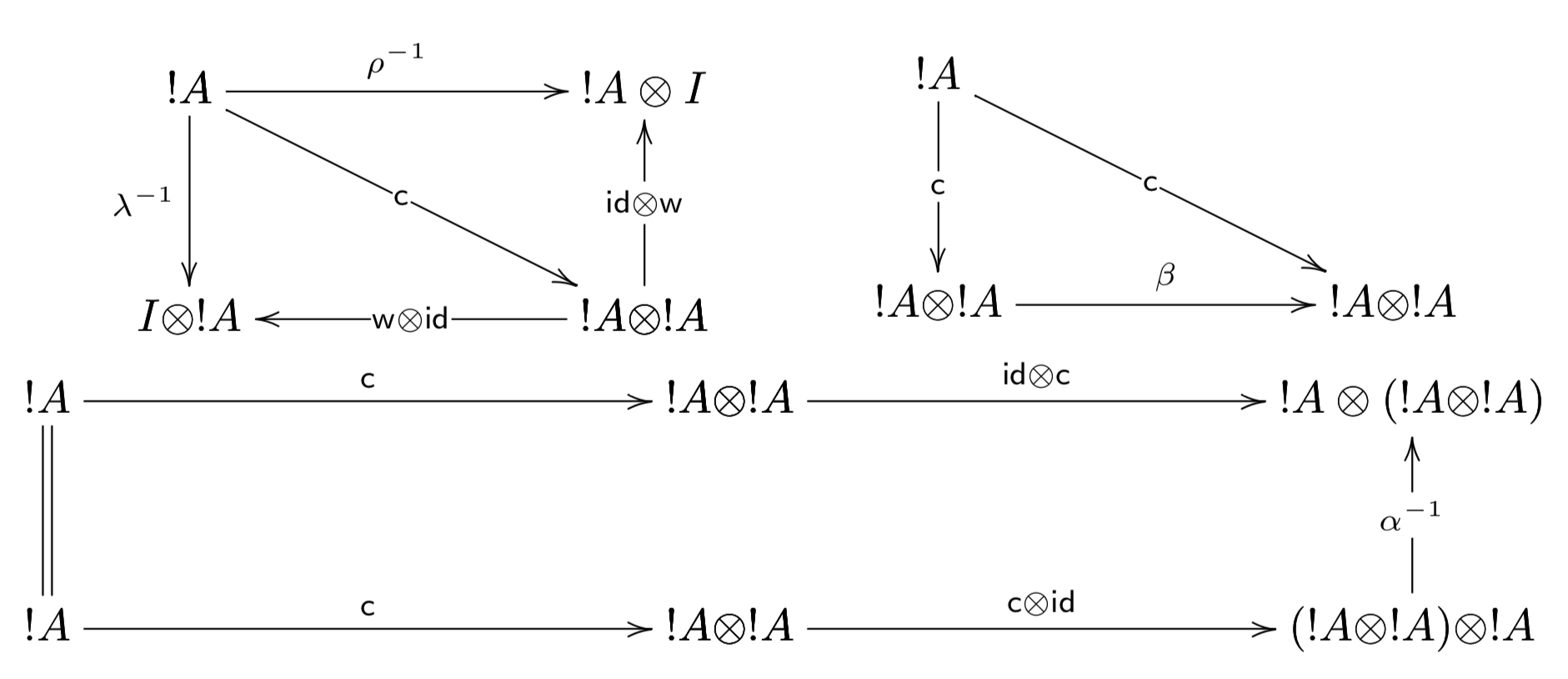• Weakening and contraction are coalgebra morphisms. That is, the following diagrams must commute: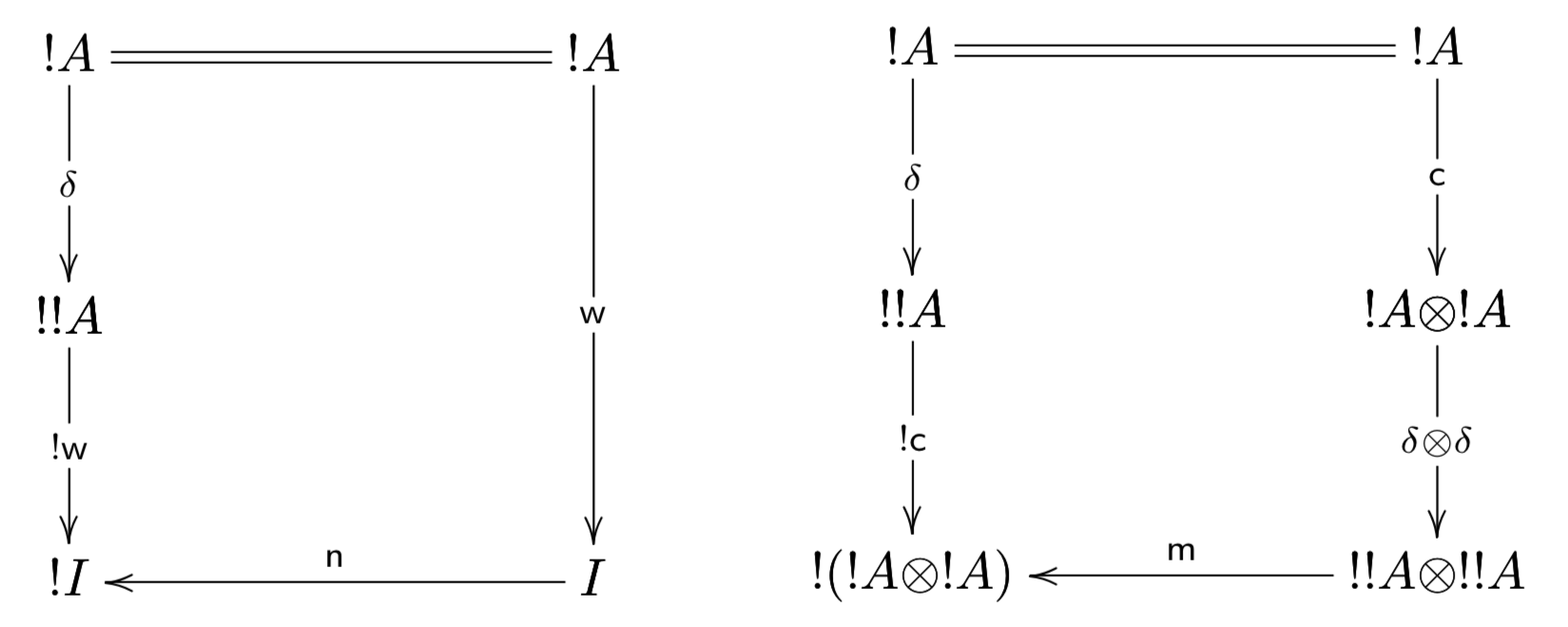• $\delta_A : !A \mto !!A$ is a comonoid morphism between the comonoids $(!A,\w,\c)$ and $(!!A,\w,\c)$. That is, the following diagrams must commute: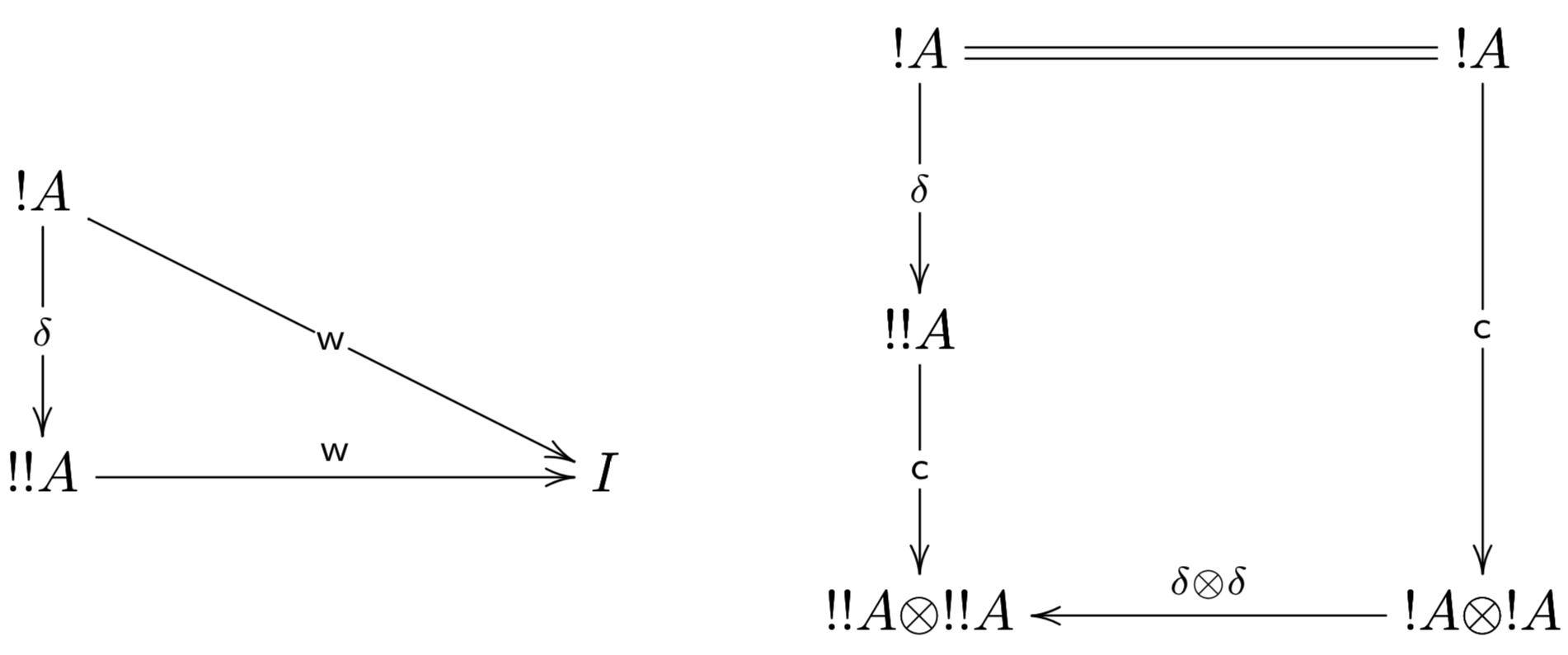All of the structure in the previous definition except for the last bullet corresponds to part 1 and part 2.a of the original definition of a linear category. The last bullet is a simplification of part 2.b. We now show that we can prove part 2.b from the assumptions in this simplified definition.

Whenever $f : (!A,\delta_A) \mto (!B,\delta_B)$ is a coalgebra morphism between free coalgebras, then it is also a comonoid morphism.

Suppose $f : (!A,\delta_A) \mto (!B,\delta_B)$ is a coalgebra morphism between free coalgebras. This assumption amounts to assuming the following diagram commutes: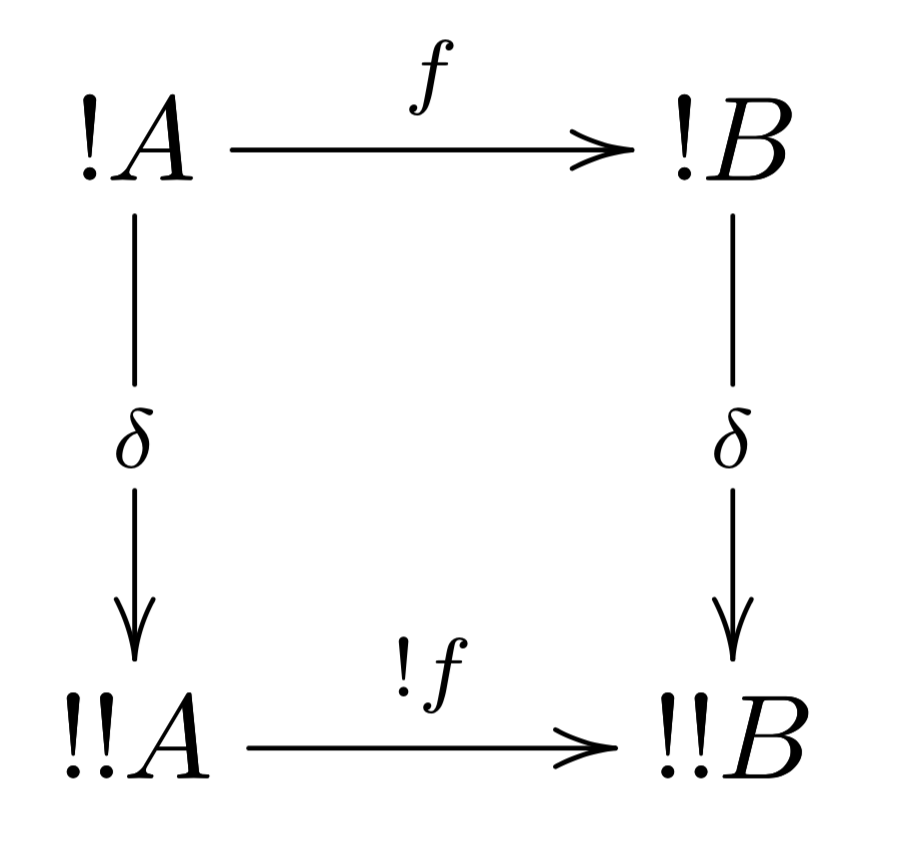It suffices to show that $f : (!A,\delta_A) \mto (!B,\delta_B)$ is also a comonoid morphism. Hence, we must show that the following diagrams commute: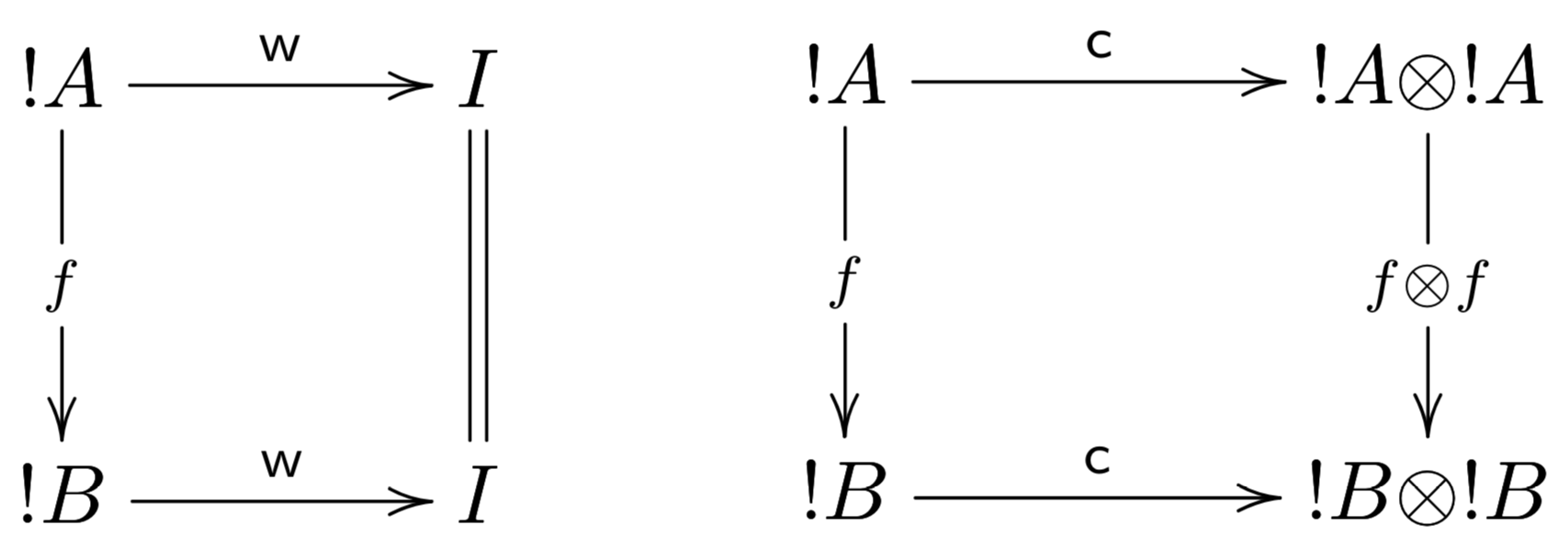The left diagram commutes, because the following expanded one does: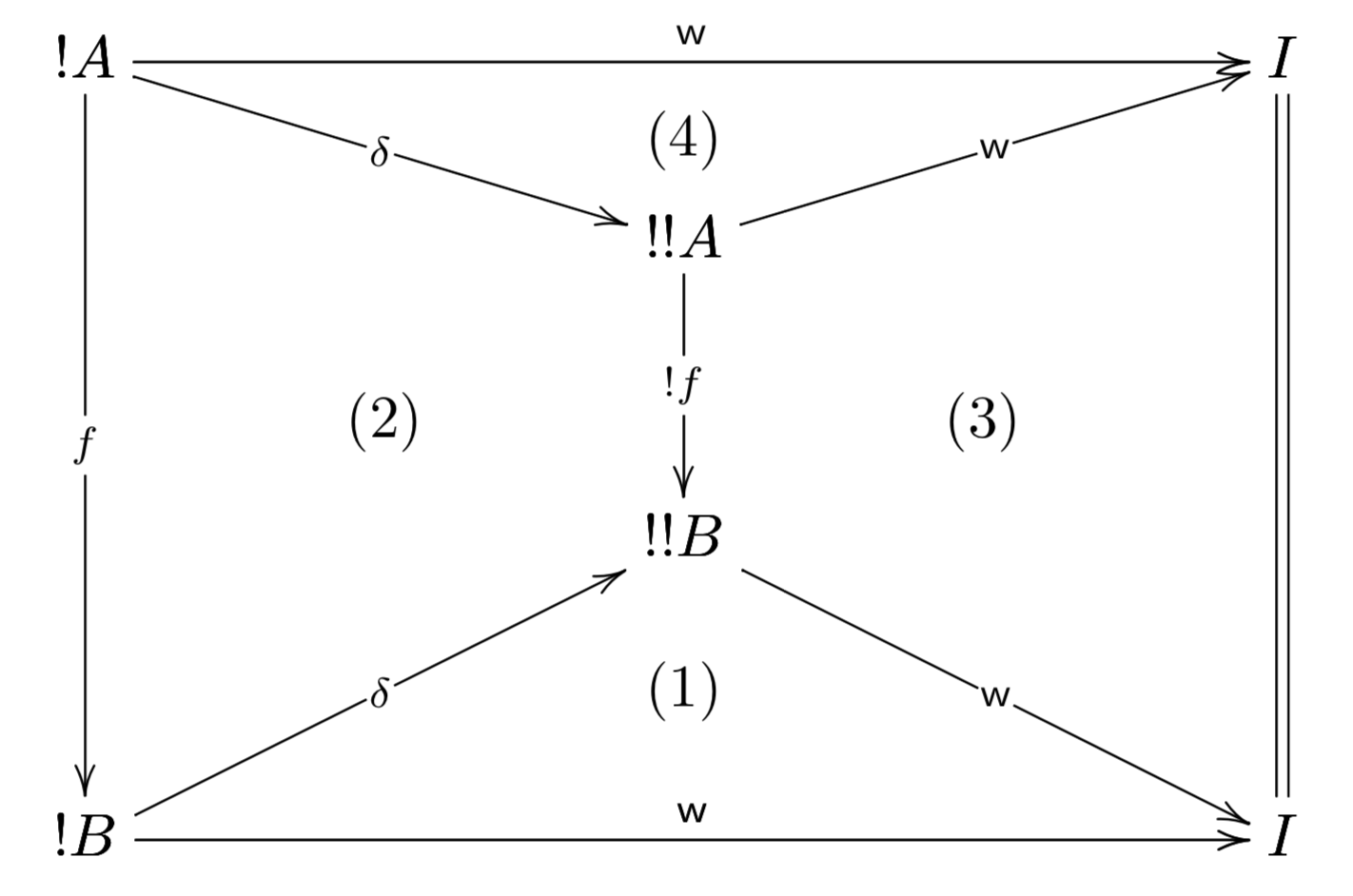Diagrams $(1)$ and $(4)$ commute because $\delta$ is a comonoid morphism, diagram $(2)$ commutes because $f$ is assumed to be a coalgebra morphism, and diagram $(3)$ commutes by naturality of $\w$. Note that we have numbered the previous diagram in the order of the necessary replacements needed when doing the same proof equationally, and we do the same for the next diagram. This should make it easier to reconstruct the proof.

The diagram for contraction commutes (the diagram on the right above), because the following expanded one does: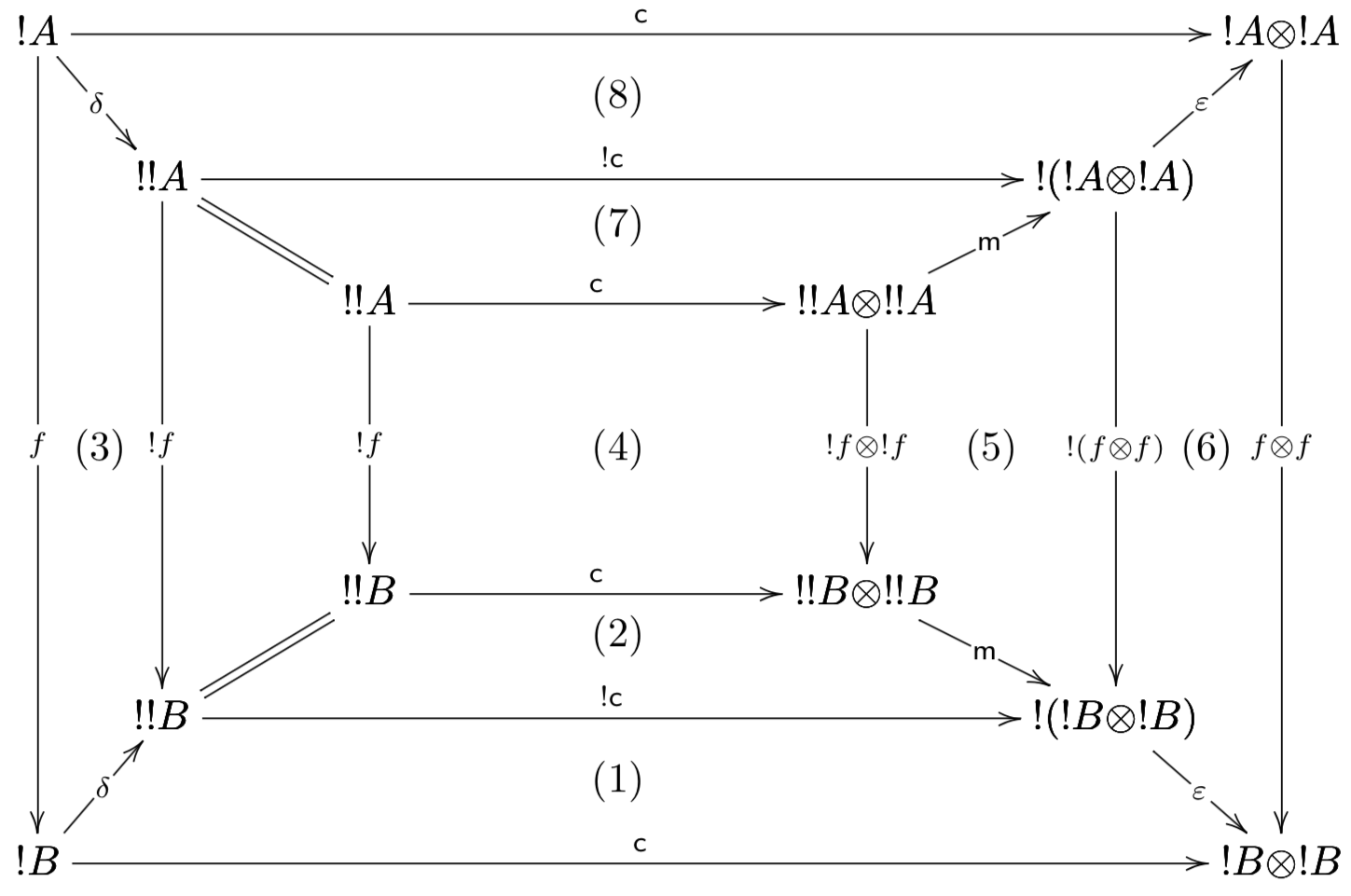Diagram $(3)$ commutes because $f$ is assumed to be a coalgebra morphism, diagram $(4)$ commutes by naturality of $\c$, diagram $(5)$ commutes by naturality of $\m$, and diagram $(6)$ commutes by naturality of $\varepsilon$.

Diagrams $(1)$ and $(8)$ commute, because the following one does: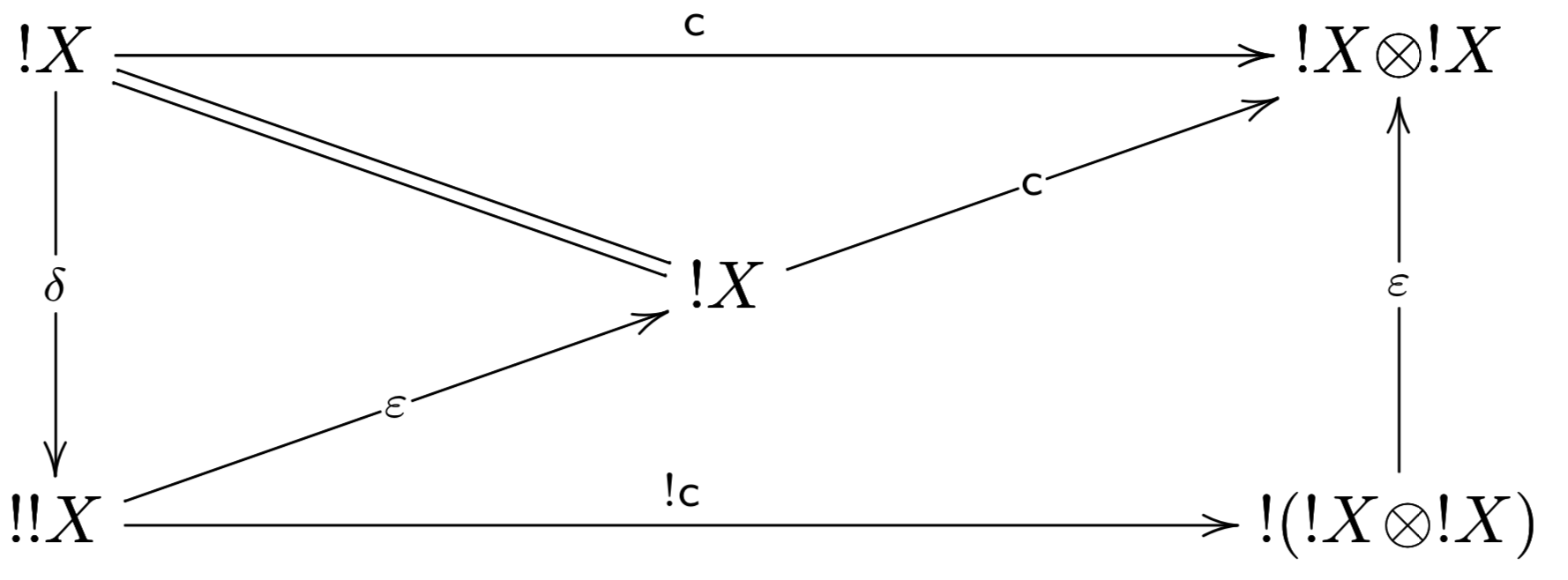The left triangle commutes, because $! : \cat{A} \mto \cat{A}$ is a comonad, and the bottom diagram commutes by naturality of $\varepsilon$.

Finally, diagrams $(2)$ and $(7)$ do not actually commute, but are parallel morphisms whose cofork is $\delta$. That is, the following diagram commutes: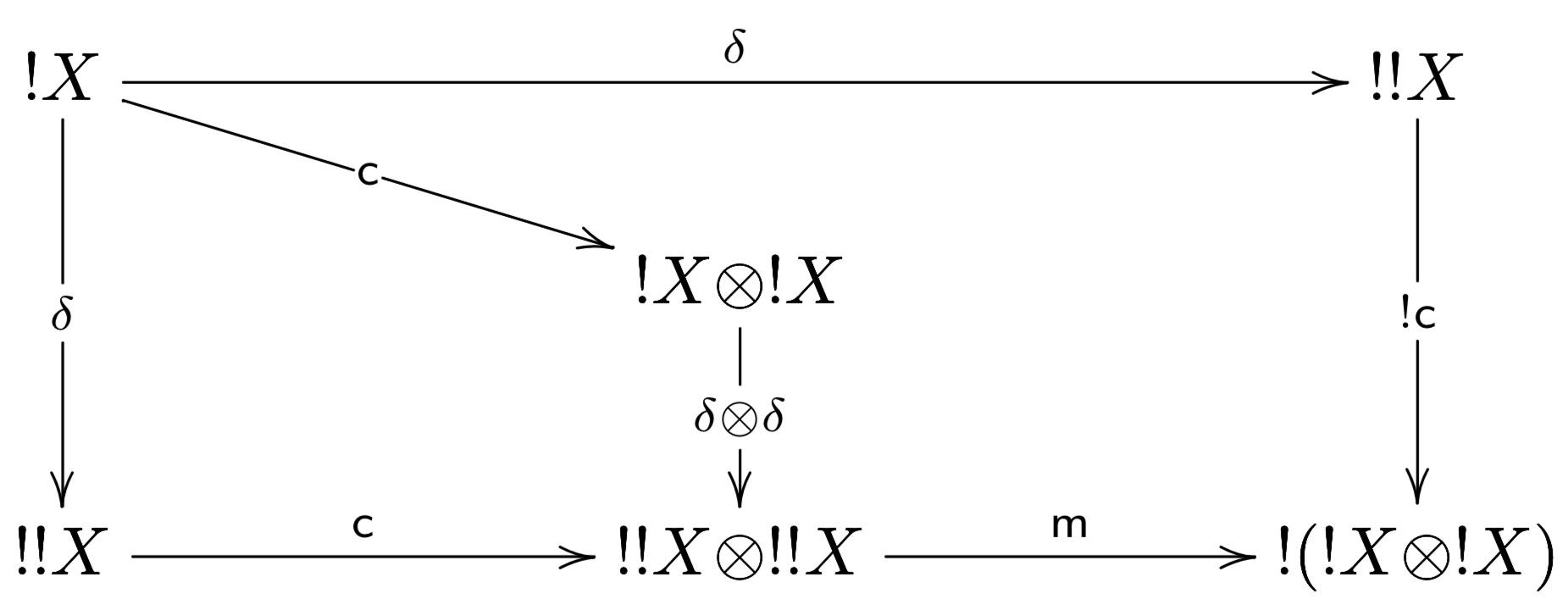The left diagram commutes because $\delta : !X \mto !!X$ is a comonoid morphism, and the right diagram commutes because weakening and contraction are coalgebra morphisms.

Therefore, the original diagram above corresponds to the following equational proof: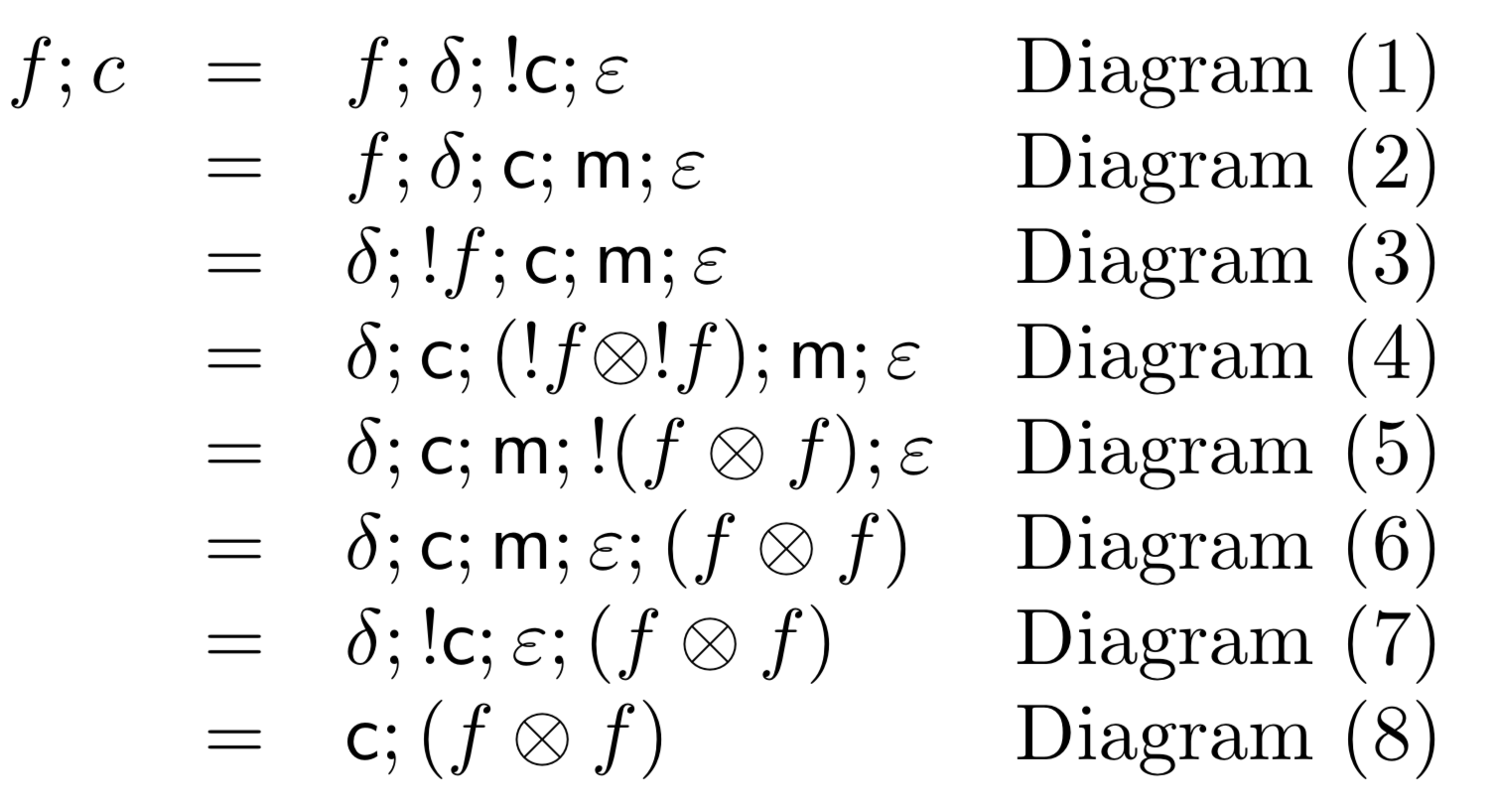## Conclusion

This was a really fun proof, but not at all obvious. Folklore results hinder scientific progress, and hinder new comers from progressing in our field. We need to be writing down these types of results so others do not have to! This is the point of research after all.

If anyone knows of a paper that writes this proof down, then I would like to know about it so I can cite it in future work. I will update this post with any citations I can find.

Updates: Jean-Simon Lemay has communicated to Damiano that this first appeared in a paper by Cocket, Seely, and Blute, but I have not had time to dig through their papers to get the precise citation yet.